Here are some effect that are possible with picFX...
I made all of them with mathematical formulas... :o)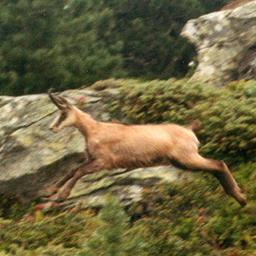This is the original picture, found on Aminet. Aminet:pix/fauna/Chamois.jpeg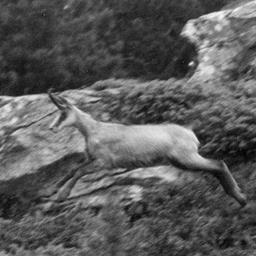black and white, each component is the mean value of the components of the source picture.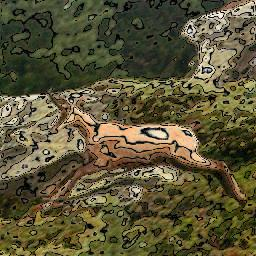Level Curves: I obtained the curves using the sine of the intensity value. Using a min(curve,picture) style function, I could mix both pictures.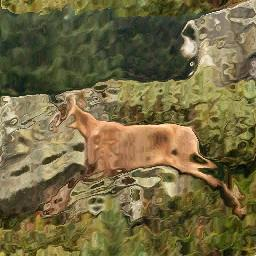Self-masking: This funny effect is what you obtain when you use the intensity of the pixel as translation mask for the same pixel :-)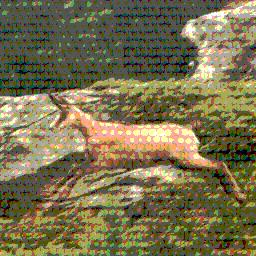kind of printing simulation: I first generated the dots using x^2+y^2, where x and y are modulo values (so that the dots repeat themselves); I then added these dots to the source picture, and substracted the modulo of the resulting picture to itself to cause colour reduction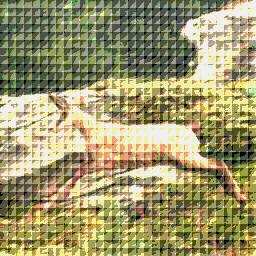The same as before, but with triangles instead of dots....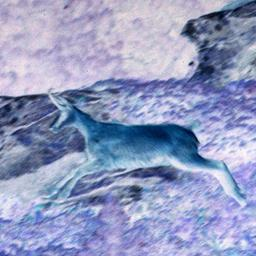negative picture... each component is the opposite of the source picture. (r(1,x,y)=255-r(0,x,y))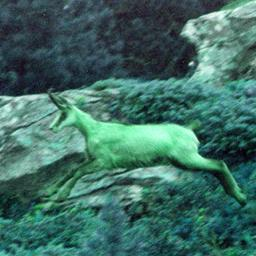Here I simply rotated the red-green-blue components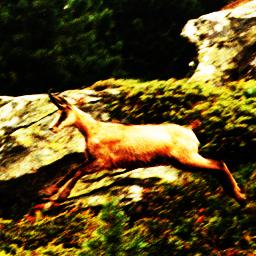A contrasted picture: I used the hyperbolic tangent to shift each of the components away from 128 (so light parts get even lighter and dark parts get even darker, and I don't need to do ugly truncation)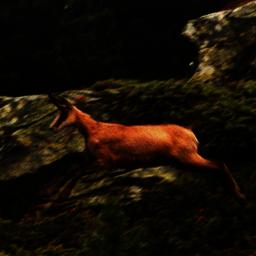This is exactely the same as the previous one, but I took the value 255 as anchor instead of 128, so that all components are shifted to zero, but a white pixel would remain white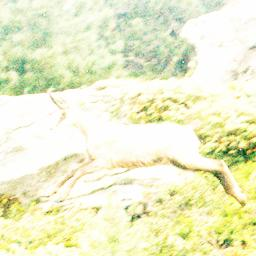The opposite of the previous one: I took zero as anchor, so that everything gets lighter...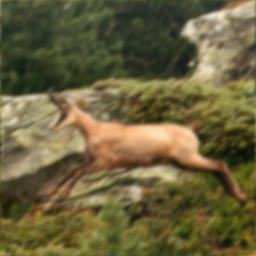Blur: This is a simple convolution operation: Each pixel is the mean value of the neighbouring pixels of the source. I made lots of blurring transformations to make the blurring realistic and spread enough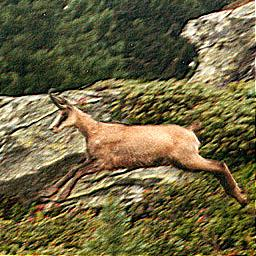Sharpen: Similar to the previous one, but each pixel is now obtained removing the neighbouring pixels to the central one, to increase colour variations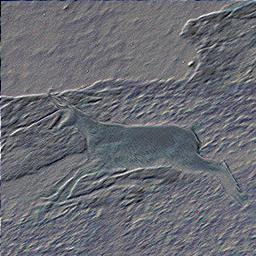Yet another convolution operator: This is in a way the difference between the sharpened picture and the source picture, with added grey.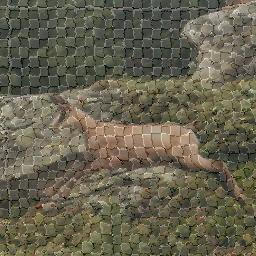Texture: This one is a bit complex; I actually used the source picture as a translation mask to that checkerboard (i.e. the red and green components of the source picture indicated how much I should move the pixels of that texture), and mixed the result to the weakened source picture.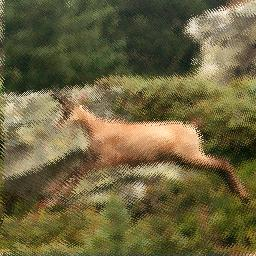Random: I first made some pseudo random picture with exponentials and other fast growing functions, to which I applied a modulo, and I then used the result as a translation mask to the source picture...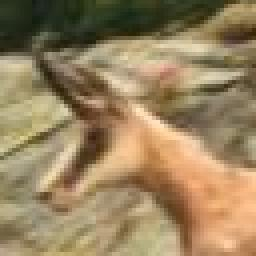Zoom: Simply read the pixels of the source dividing the x and y variables... I was too lazy to do interpolation, but it's not hard to do ;-)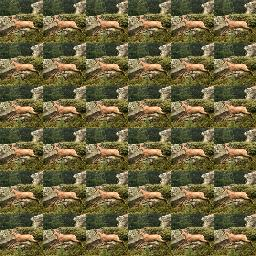Tiled: The exact reverse of the previous one, I multiplied the variable to reduce the size of the picture. (Modulo is applied automatically when we attempt to read a pixel outside of the domain of the source picture)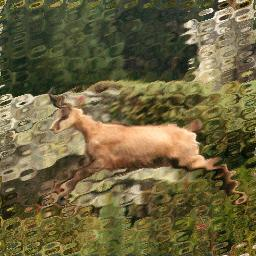Glass: Very easy to do. I used the product of two sines as a translation mask. I also multiplied it by the distance to the center, so that the effect is stronger at the border...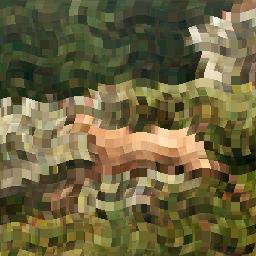Mosaic: I first did the squares with something like x-(x%16), which is a function that looks like stairs. So reading the source picture with such coordinate produces these kind of tiles, as we read the same pixel on quite a wide area. I then transformed it using some simple sine functions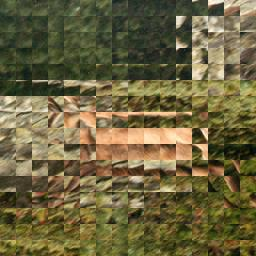Inverted: Each little block is a central symmetry of the source's. I obtained this with the same equation y-(x%16) as the previous one, but doubled the modulo. Then, instead of reading always the same pixel, inside one block, we read backward...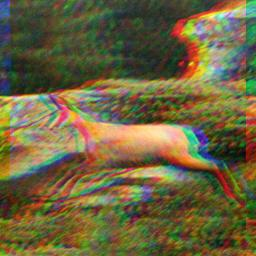Here I read the three components of the source, with a ten pixel shift for red and blue...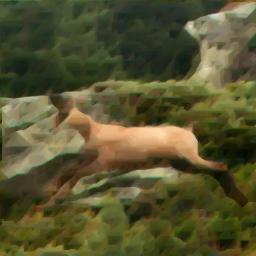Smoothed: I repeatedly performed the following operation: For each pixel, replace it by the darkest (lightest) neighbouring pixel. After some time, this has the effect of removing peaks in the picture, thus giving it that smoothed look. (The triangular shapes you see in some place are due to the choice of these neiboughring pixels)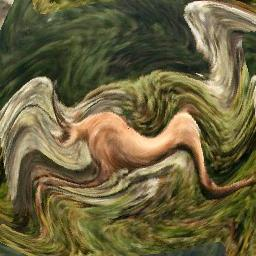In this one, I made the pixels turn around the center (Actually, they don't exactely turn, but instead go some distance straight in a direction perpendicular to the center). The amount of displacement is some sine function of the distance to the center. To avoid having huge equations, I calculated that distance in a separate project, and then referenced it every where I needed